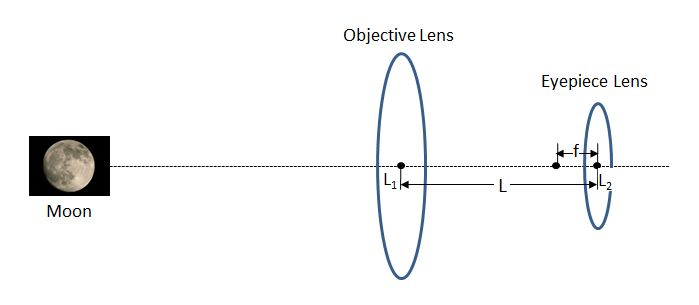# Keplerian TelescopeThe Keplerian Telescope, invented by Johannes Kepler in $1611,$ is an improvement on Galileo's design. It uses a convex lens as the eyepiece instead of Galileo's concave one. The above is a schematic design of the Keplerian Telescope, where the center of the objective and eyepiece lenses are $L_1$ and $L_2$, respectively. When the distance between them is $L$, the image of the moon becomes the sharpest. If the focal length of the eyepiece lens is $f,$ then which of the following statements is NOT correct?

a) The focal length of the objective lens must be larger than $L.$

b) The image shown by the eyepiece lens is a virtual image.

c) The distance between the image created by the objective lens and the center of the eyepiece lens is smaller than $f.$

×